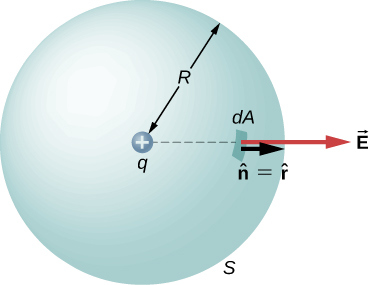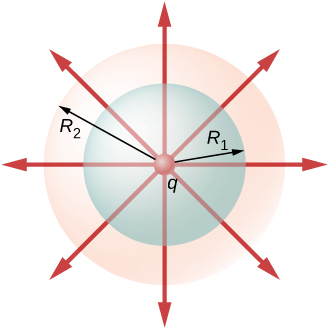# 6.2 Explaining gauss’s law

 Page 1 / 4
By the end of this section, you will be able to:
• State Gauss’s law
• Explain the conditions under which Gauss’s law may be used
• Apply Gauss’s law in appropriate systems

We can now determine the electric flux through an arbitrary closed surface due to an arbitrary charge distribution. We found that if a closed surface does not have any charge inside where an electric field line can terminate, then any electric field line entering the surface at one point must necessarily exit at some other point of the surface. Therefore, if a closed surface does not have any charges inside the enclosed volume, then the electric flux through the surface is zero. Now, what happens to the electric flux if there are some charges inside the enclosed volume? Gauss’s law gives a quantitative answer to this question.

To get a feel for what to expect, let’s calculate the electric flux through a spherical surface around a positive point charge q , since we already know the electric field in such a situation. Recall that when we place the point charge at the origin of a coordinate system, the electric field at a point P that is at a distance r from the charge at the origin is given by

${\stackrel{\to }{\text{E}}}_{P}=\frac{1}{4\pi {\epsilon }_{0}}\phantom{\rule{0.2em}{0ex}}\frac{1}{{r}^{2}}\stackrel{^}{\text{r}},$

where $\stackrel{^}{\text{r}}$ is the radial vector from the charge at the origin to the point P. We can use this electric field to find the flux through the spherical surface of radius r , as shown in [link] .A closed spherical surface surrounding a point charge q .

Then we apply $\text{Φ}={\int }_{S}\stackrel{\to }{E}·\stackrel{^}{n}\phantom{\rule{0.2em}{0ex}}dA$ to this system and substitute known values. On the sphere, $\stackrel{^}{n}=\stackrel{^}{r}$ and $r=R$ , so for an infinitesimal area dA ,

$d\text{Φ}=\stackrel{\to }{E}·\stackrel{^}{n}\phantom{\rule{0.2em}{0ex}}dA=\frac{1}{4\pi {\epsilon }_{0}}\phantom{\rule{0.2em}{0ex}}\frac{q}{{R}^{2}}\stackrel{^}{r}·\stackrel{^}{r}\phantom{\rule{0.2em}{0ex}}dA=\frac{1}{4\pi {\epsilon }_{0}}\phantom{\rule{0.2em}{0ex}}\frac{q}{{R}^{2}}\phantom{\rule{0.2em}{0ex}}dA.$

We now find the net flux by integrating this flux over the surface of the sphere:

$\text{Φ}=\frac{1}{4\pi {\epsilon }_{0}}\phantom{\rule{0.2em}{0ex}}\frac{q}{{R}^{2}}{\oint }_{S}\phantom{\rule{0.2em}{0ex}}dA=\frac{1}{4\pi {\epsilon }_{0}}\phantom{\rule{0.2em}{0ex}}\frac{q}{{R}^{2}}\left(4\pi {R}^{2}\right)=\frac{q}{{\epsilon }_{0}}.$

where the total surface area of the spherical surface is $4\pi {R}^{2}.$ This gives the flux through the closed spherical surface at radius r as

$\text{Φ}=\frac{q}{{\epsilon }_{0}}.$

A remarkable fact about this equation is that the flux is independent of the size of the spherical surface. This can be directly attributed to the fact that the electric field of a point charge decreases as $1\text{/}{r}^{2}$ with distance, which just cancels the ${r}^{2}$ rate of increase of the surface area.

## Electric field lines picture

An alternative way to see why the flux through a closed spherical surface is independent of the radius of the surface is to look at the electric field lines. Note that every field line from q that pierces the surface at radius ${R}_{1}$ also pierces the surface at ${R}_{2}$ ( [link] ).Flux through spherical surfaces of radii R 1 and R 2 enclosing a charge q are equal, independent of the size of the surface, since all E -field lines that pierce one surface from the inside to outside direction also pierce the other surface in the same direction.

Therefore, the net number of electric field lines passing through the two surfaces from the inside to outside direction is equal. This net number of electric field lines, which is obtained by subtracting the number of lines in the direction from outside to inside from the number of lines in the direction from inside to outside gives a visual measure of the electric flux through the surfaces.

#### Questions & Answers

What mass of steam of 100 degree celcius must be mixed with 150g of ice at 0 degree celcius, in a thermally insulated container, to produce liquid water at 50 degree celcius
sorry I dont know
Bamidele
thank you
Emmanuel
What is the pressure?
SHREESH
To convert 0°C ice to 0°c water. Q=M*s=150g*334J/g=50100 J.......... Now 0° water to 50° water... Q=M*s*dt=150g*4.186J/g*50= 31395 J....... Which adds upto 81495 J..... This is amount of heat the steam has to carry. 81495= M *s=M*2230J/g..therefore.....M=36.54g of steam
SHREESH
This is at 1 atm
SHREESH
If there is change in pressure u can refer to the steam table ....
SHREESH
instrument for measuring highest temperature of a body is?
Thermometer
Umar
how does beryllium decay occur
Photon?
Umar
state the first law of thermodynamics
Its state that "energy can neither be created nor destroyed but can be transformed from one form to another. "
Ayodamola
what about the other laws can anyone here help with it please
Sandy
The second law of thermodynamics states that the entropy of any isolated system always increases. The third law of thermodynamics states that the entropy of a system approaches a constant value as the temperature approaches absolute zero.
sahil
The first law is very simple to understand by its equation. The law states that "total energy in thermodynamic sytem is always constant" i.e d¶=du+dw where d¶=total heat du=internal energy dw=workdone... PLEASE REFER TO THE BOOKS FOR MORE UNDERSTANDING OF THE CONCEPT.
Elia
what is distance.?
what is physics?
Ali
Physics is a scientific phenomenon that deals with matter and its properties
Ayodamola
physics is the study of nature and science
John
Chater1to7
min
Physics is branch of science which deals with the study of matters in relation with energy.
Elia
What is differential form of Gauss's law?
help me out on this question the permittivity of diamond is 1.46*10^-10.( a)what is the dielectric of diamond (b) what its susceptibility
a body is projected vertically upward of 30kmp/h how long will it take to reach a point 0.5km bellow e point of projection
i have to say. who cares. lol. why know that t all
Jeff
is this just a chat app about the openstax book?
kya ye b.sc ka hai agar haa to konsa part
what is charge quantization
it means that the total charge of a body will always be the integral multiples of basic unit charge ( e ) q = ne n : no of electrons or protons e : basic unit charge 1e = 1.602×10^-19
Riya
is the time quantized ? how ?
Mehmet
What do you meanby the statement,"Is the time quantized"
Mayowa
Can you give an explanation.
Mayowa
there are some comment on the time -quantized..
Mehmet
time is integer of the planck time, discrete..
Mehmet
planck time is travel in planck lenght of light..
Mehmet
it's says that charges does not occur in continuous form rather they are integral multiple of the elementary charge of an electron.
Tamoghna
it is just like bohr's theory. Which was angular momentum of electron is intral multiple of h/2π
determine absolute zero
The properties of a system during a reversible constant pressure non-flow process at P= 1.6bar, changes from constant volume of 0.3m³/kg at 20°C to a volume of 0.55m³/kg at 260°C. its constant pressure process is 3.205KJ/kg°C Determine: 1. Heat added, Work done, Change in Internal Energy and Change in Enthalpy
U can easily calculate work done by 2.303log(v2/v1)
Abhishek
Amount of heat added through q=ncv^delta t
Abhishek
Change in internal energy through q=Q-w
Abhishek
please how do dey get 5/9 in the conversion of Celsius and Fahrenheit
what is copper loss
this is the energy dissipated(usually in the form of heat energy) in conductors such as wires and coils due to the flow of current against the resistance of the material used in winding the coil.
HenryByBy David Martin